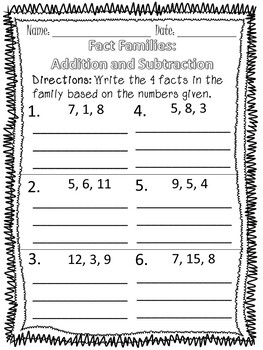# Addition and Subtraction Fact FamiliesSubject
Resource Type
File Type

PDF

(2 MB|27 pages)
Product Rating
Standards
• Product Description
• StandardsNEW

This is a useful set of worksheets that help students learn to solve problems using their knowledge of addition and subtraction fact families.

This pack contains 25 black and white worksheets. They are designed to help students solve problems involving addition and subtraction fact families by tackling them in several different ways. All worksheets are designed as no prep printables, just print and go! Details of worksheets are listed below.

Fill in the Blank Fact Family-10 worksheets

Write the Missing Facts-10 worksheets (2 different ways)

Write all the Facts Given the Numbers-5 worksheets

Check out my other math bundles!

Add and subtract within 20, demonstrating fluency for addition and subtraction within 10. Use strategies such as counting on; making ten (e.g., 8 + 6 = 8 + 2 + 4 = 10 + 4 = 14); decomposing a number leading to a ten (e.g., 13 - 4 = 13 - 3 - 1 = 10 - 1 = 9); using the relationship between addition and subtraction (e.g., knowing that 8 + 4 = 12, one knows 12 - 8 = 4); and creating equivalent but easier or known sums (e.g., adding 6 + 7 by creating the known equivalent 6 + 6 + 1 = 12 + 1 = 13).
Total Pages
27 pages
N/A
Teaching Duration
N/A
Report this Resource to TpT
Reported resources will be reviewed by our team. Report this resource to let us know if this resource violates TpT’s content guidelines.